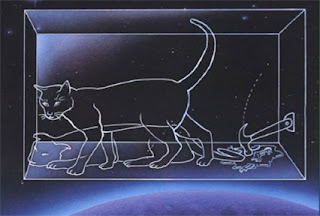## Wednesday, March 21, 2007

### The measurement problem in quantum mechanicsThe measurement problem in quantum mechanics is fundamentally mis-understood, and, as a consequence, the most popular solutions to it are mis-conceived. The measurement problem is as follows: whilst an isolated microscopic system evolves continuously and deterministically, in accordance with the Schrodinger equation, when that system is subjected to a measurement-like interaction, the state of the system collapses discontinuously and non-deterministically into an 'eigenstate' of the quantity being measured, a state in which that quantity has a definite value. The state of a system in quantum mechanics is often called the wave-function, hence this is referred to as the collapse of the wave-function. 'Schrodinger's cat' was ostensibly devised as a reductio ad absurdum, to demonstrate that the consequences of not accepting the collapse of the wave-function are inconsistent with empirical experience: we do not observe macroscopic superpositions, such as that between a dead-cat state and a live-cat state, hence a measurement-like interaction must collapse the state of the system being measured, rather than entangle the macroscopic, measurement system in the superposed state of the microscopic system. The measurement problem, then, is often posed in the following terms: are there really two distinct types of evolution process in quantum mechanics, or is the collapse of the wave-function just an apparent collapse?

The measurement problem, however, is really just one facet of a more general question: given that a macroscopic system is composed of nothing else but microscopic systems satisfying the principles of quantum theory, how does the classical, macroscopic world emerge from the quantum microscopic world? At this juncture, it is crucial to appreciate the distinction between the kinematics and the dynamics of a theory. The kinematics provide a characterisation of a system at a moment in time, whilst the dynamics specify how the state of a system evolves in time. Now, the kinematics of classical physics are fundamentally inconsistent with the kinematics of quantum physics. In classical physics, every state specifies a simultaneous, precise value for every quantity of a system, and when we observe a macroscopic system, we observe it to have simultaneous definite values for all its quantities. In contrast, in quantum physics there is no state which specifies a simultaneous precise value for all the quantities of a system; the values of some quantities are specified, others receive only a probability distribution over their possible values.

Now, the measurement problem is really just a manifestation of this fundamental inconsistency between the kinematics of classical and quantum physics. Contrary to the popular belief, even amongst physicists and philosophers of science, the measurement problem is not a problem for the dynamics of quantum theory. The point about a measurement-like interaction is that to measure the value of a quantity on a microscopic system, the outcome of a microscopic event must be amplified onto macroscopic length-scales. The existence of such amplificatory processes demonstrates that the classical, macroscopic world cannot be insulated from the kinematics of the quantum world. The amplificatory process demonstrates the inconsistency between quantum and classical kinematics, but the amplificatory process itself is not the issue.

Physicists generally believe these days that something called 'decoherence' resolves the measurement problem in quantum mechanics. This is an attempt to solve the measurement problem by tinkering with evolution processes, and it is therefore doomed to fail:

Given a collection of quantum states, one can either form another state which is a superposition of those states, or one can form another state which is a 'mixture' of those states. The probability distributions of a superposed state are different from those of a mixture because of 'interference terms'. Whilst the probabilities in a mixture can be interpreted as expressions of ignorance, the probabilities in a superposition are interpreted as expressions of objective indeterminacy. For a system in a mixed state, one can say that the system actually resides in one of the states, but one is simply ignorant as to which one; for a system in a superposition, one cannot say that the system actually resides in one of the superposed states. The aim of decoherence is to show that under a measurement-like interaction, the interference terms tend to zero, hence the microscopic superposition evolves into a macroscopic mixture. This approach is doomed to failure because, even if one could manipulate the final state of the measurement apparatus (or cat) into one of the quantum states from the original superposition, the kinematics of quantum theory would still apply: that final quantum state would not specify simultaneous, definite values for all the quantities of the measurement apparatus, yet simultaneous definite values are exactly what one observes the measurement apparatus to possess. In quantum theory, every quantity has a 'conjugate' quantity, such that if the current state makes one quantity definite, the conjugate quantity will be indefinite. Position and momentum, for example, are conjugate quantities. So even if one could manipulate the final state of the measurement apparatus into a state which assigns a definite value to the quantity which indicates the result of the measurement, that indicator variable must have a conjugate variable which will be indefinite in that state. Tinkering with the dynamics of measurement processes will not solve the fundamental problem, which is the inconsistency between the kinematics of quantum theory and the kinematics of classical theory.